Next: 5.1 Conventional Approach Up: Dissertation Otmar Ertl Previous: 4.10.3 Benchmarks

# 5. Surface Rate Calculation

The simulation of surface evolution requires the calculation of the surface velocity at every time step. For advanced models, the surface velocity depends on the particle transport. According to the surface kinetics model described in Section 2.3, the surface velocity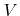is assumed to be a function of a certain number of surface rates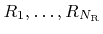(2.25). This chapter is devoted to the calculation of these surface rates under the assumption of ballistic particle transport within the feature-scale region, as described by (2.14). Particles entering the feature-scale region through the source plane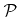follow the given arriving flux distribution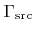. Particle reemission, modelled by the reemission probability function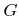(2.15), must also be incorporated. In summary the problem states as follows:

• Given:,for all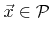, andfor all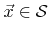.
• Required:(in order to calculate) for all.
Two completely different approaches for solving this problem are presented in the following sections.

SubsectionsNext: 5.1 Conventional Approach Up: Dissertation Otmar Ertl Previous: 4.10.3 Benchmarks

Otmar Ertl: Numerical Methods for Topography Simulation